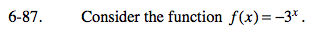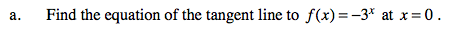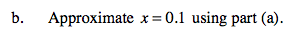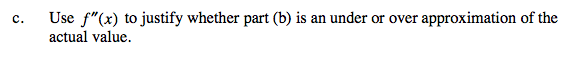### Home > CALC > Chapter 6 > Lesson 6.3.1 > Problem6-87

6-87.Evaluate f '(0) to find the slope, and evaluate f(0) to find the y-intercept. Use the point-slope formula to create the equation of the tangent line.Use the tangent line at x = 0 to evaluate x = 0.1. This will approximate f(0.1). Why approximate?
Since a tangent is a linear equation, it is easier to evaluate than f(x).A concave up graph has tangent lines that are below the curve. Sketch it, you will see.
And a concave down graph has tangent lines that are above the curve.

Overestimate since f ''(0) = −(ln 3)2 · 30 = −(ln 3)2 < 0